# Lateral Controller Stanley

Control steering angle of vehicle for path following by using Stanley method

Since R2018b

•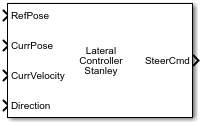Libraries:
Automated Driving Toolbox / Vehicle Control

## Description

The Lateral Controller Stanley block computes the steering angle command, in degrees, that adjusts the current pose of a vehicle to match a reference pose, given the vehicle's current velocity and direction. The controller computes this command using the Stanley method , whose control law is based on both a kinematic and dynamic bicycle model. To change between models, use the Vehicle model parameter.

• The kinematic bicycle model is suitable for path following in low-speed environments such as parking lots, where inertial effects are minimal.

• The dynamic bicycle model is suitable for path following in high-speed environments such as highways, where inertial effects are more pronounced. This vehicle model provides additional parameters that describe the dynamics of the vehicle.

## Ports

### Input

expand all

Reference pose, specified as an [x, y, Θ] vector. x and y are in meters, and Θ is in degrees.

x and y specify the reference point to steer the vehicle toward. Θ specifies the orientation angle of the path at this reference point and is positive in the counterclockwise direction.

• For a vehicle in forward motion, the reference point is the point on the path that is closest to the center of the vehicle's front axle.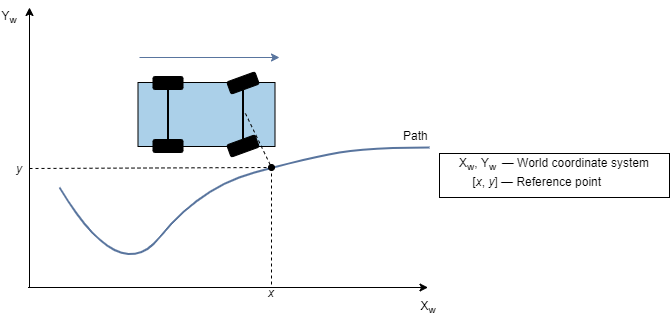• For a vehicle in reverse motion, the reference point is the point on the path that is closest to the center of the vehicle's rear axle.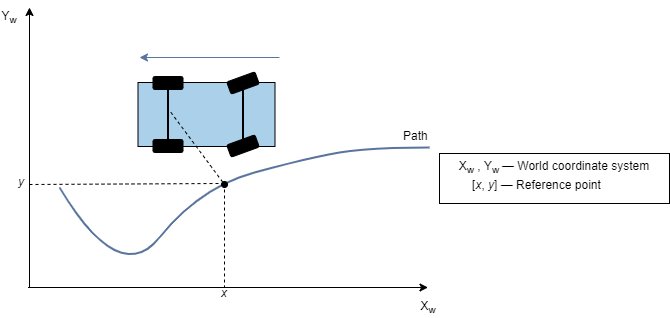Data Types: `single` | `double`

Current pose of the vehicle, specified as an [x, y, Θ] vector. x and y are in meters, and Θ is in degrees.

x and y specify the location of the vehicle, which is defined as the center of the vehicle's rear axle.

Θ specifies the orientation angle of the vehicle at location (x,y) and is positive in the counterclockwise direction.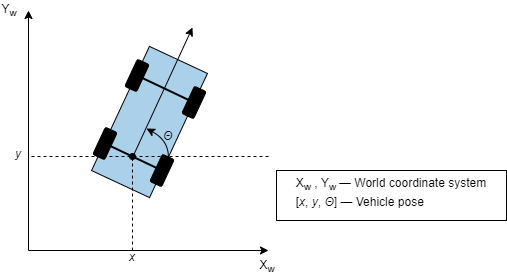For more details on vehicle pose, see Coordinate Systems in Automated Driving Toolbox.

Data Types: `single` | `double`

Current longitudinal velocity of the vehicle, specified as a real scalar. Units are in meters per second.

• If the vehicle is in forward motion, then this value must be greater than 0.

• If the vehicle is in reverse motion, then this value must be less than 0.

• A value of 0 represents a vehicle that is not in motion.

Data Types: `single` | `double`

Driving direction of the vehicle, specified as `1` for forward motion or `-1` for reverse motion. The driving direction determines the position error and angle error used to compute the steering angle command. For more details, see Algorithms.

Curvature of the path at the reference point, in radians per meter, specified as a real scalar.

• For a vehicle in forward motion, the reference point is the point on the path that is closest to the center of the vehicle's front axle.• For a vehicle in reverse motion, the reference point is the point on the path that is closest to the center of the vehicle's rear axle.You can obtain the curvature of a path from the Curvatures output port of a Path Smoother Spline block. You can also obtain curvatures of lane boundaries from the output lane boundary structures of a Scenario Reader block.

#### Dependencies

To enable this port, set Vehicle model to `Dynamic bicycle model`.

Current yaw rate of the vehicle, in degrees per second, specified as a real scalar. The current yaw rate is the rate of change in the angular velocity of the vehicle.

#### Dependencies

To enable this port, set Vehicle model to `Dynamic bicycle model`.

Current steering angle of the vehicle, in degrees, specified as a real scalar. This value is positive in the counterclockwise direction.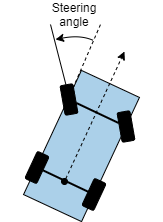For more details, see Coordinate Systems in Automated Driving Toolbox.

#### Dependencies

To enable this port, set Vehicle model to `Dynamic bicycle model`.

### Output

expand all

Steering angle command, in degrees, returned as a real scalar. This value is positive in the counterclockwise direction.For more details, see Coordinate Systems in Automated Driving Toolbox.

## Parameters

expand all

Select the type of vehicle model to set the Stanley method control law used by the block.

• `Kinematic bicycle model` — Kinematic bicycle model for path following in low-speed environments such as parking lots, where inertial effects are minimal

• `Dynamic bicycle model` — Dynamic bicycle model for path following in high-speed environments such as highways, where inertial effects are more pronounced

Position gain of the vehicle when it is in forward motion, specified as a positive scalar. This value determines how much the position error affects the steering angle. Typical values are in the range [1, 5]. Increase this value to increase the magnitude of the steering angle.

Position gain of the vehicle when it is in reverse motion, specified as a positive scalar. This value determines how much the position error affects the steering angle. Typical values are in the range [1, 5]. Increase this value to increase the magnitude of the steering angle.

Yaw rate feedback gain, specified as a nonnegative real scalar. This value determines how much weight is given to the current yaw rate of the vehicle when the block computes the steering angle command.

#### Dependencies

To enable this parameter, set Vehicle model to `Dynamic bicycle model`.

Steering angle feedback gain, specified as a nonnegative real scalar. This value determines how much the difference between the current steering angle command, SteerCmd, and the current steering angle, CurrSteer, affects the next steering angle command.

#### Dependencies

To enable this parameter, set Vehicle model to `Dynamic bicycle model`.

Distance between the front and rear axle of the vehicle, in meters, specified as a real scalar. This value applies only when the vehicle is in forward motion, that is, when the Direction input port is `1`.

#### Dependencies

To enable this parameter, set Vehicle model to `Kinematic bicycle model`.

Vehicle mass, in kilograms, specified as a positive real scalar.

#### Dependencies

To enable this parameter, set Vehicle model to `Dynamic bicycle model`.

Longitudinal distance from the vehicle's center of mass to its front wheel axle, in meters, specified as a positive real scalar.

#### Dependencies

To enable this parameter, set Vehicle model to `Dynamic bicycle model`.

Longitudinal distance from the vehicle's center of mass to its rear wheel axle, in meters, specified as a positive real scalar.

#### Dependencies

To enable this parameter, set Vehicle model to `Dynamic bicycle model`.

Cornering stiffness of front tires, in Newtons per radian, specified as a positive real scalar.

#### Dependencies

To enable this parameter, set Vehicle model to `Dynamic bicycle model`.

Maximum allowed steering angle of the vehicle, in degrees, specified as a real scalar in the range (0, 180).

The output from the SteerCmd port is saturated to the range [–M, M], where M is the value of the Maximum steering angle (deg) parameter.

• Values below –M are set to –M.

• Values above M are set to M.

## Tips

• You can switch between bicycle models as the vehicle environment changes. Add two Lateral Controller Stanley blocks to a variant subsystem and specify a different bicycle model for each block. For an example, see Lateral Control Tutorial.

## Algorithms

To compute the steering angle command, the controller minimizes the position error and the angle error of the current pose with respect to the reference pose. The driving direction of the vehicle determines these error values.

When the vehicle is in forward motion (Direction parameter is `1`):

• The position error is the lateral distance from the center of the front axle to the reference point on the path.

• The angle error is the angle of the front wheel with respect to reference path.

When the vehicle is in reverse motion (Direction parameter is `-1`):

• The position error is the lateral distance from the center of the rear axle to the reference point on the path.

• The angle error is the angle of the rear wheel with respect to reference path.

For details on how the controller minimizes these errors for kinematic and dynamic bicycle models, see .

 Hoffmann, Gabriel M., Claire J. Tomlin, Michael Montemerlo, and Sebastian Thrun. "Autonomous Automobile Trajectory Tracking for Off-Road Driving: Controller Design, Experimental Validation and Racing." American Control Conference. 2007, pp. 2296–2301. doi:10.1109/ACC.2007.4282788